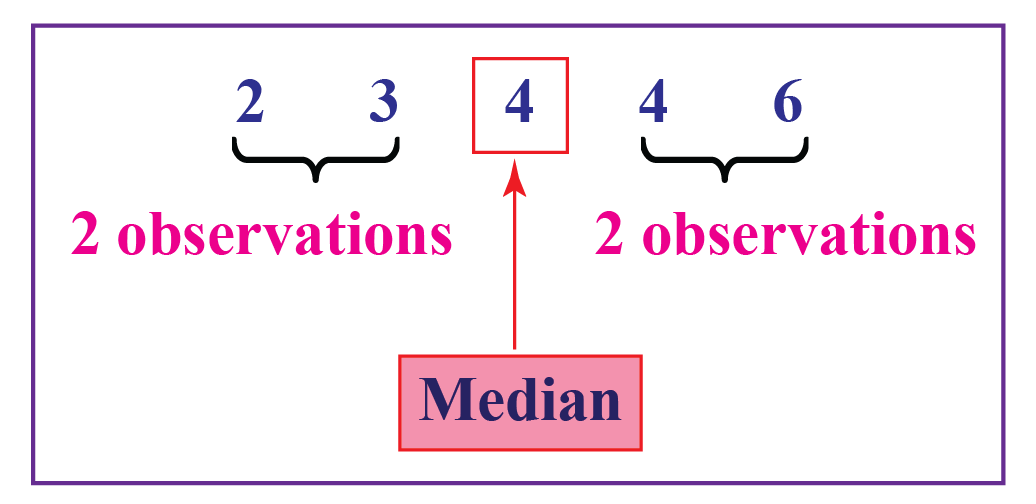# Mean Median Mode

Published:

This post covers Introduction to descriptive statistics.

# Questions

## Mean

$\bar{x} = \frac{\Sigma x_if_i}{\Sigma f_i}$

### Raw Data

• A cricketer’s scores in five ODI matches are as follows: $12, 34, 45, 50, 24$. Find the mean score.
• $\bar{x} = 33$
• If the heights of 5 people are $142 cm, 150 cm, 149 cm, 156 cm, and 153 cm$. Find the mean height.
• $\bar{x} = 150$

### Tabular (Frequency Distribution) Form

• Find the mean of the following distribution:

x4691015
f5101078

$\bar{x} = \frac{\Sigma x_if_i}{\Sigma f_i} = 9$

• The following table indicates the data on the number of patients visiting a hospital in a month. Find the average number of patients visiting the hospital in a day.

Number of PatientsNumber of days visiting hospital
0-102
10-206
20-309
30-407
40-504
50-602

$class~mark = x_i = \frac{lower+upper}{2}$

$\bar{x} = \frac{\Sigma x_if_i}{\Sigma f_i} = 28.67$

• Challenging:

• Let the mean of $x_1,~ x_2,~ x_3,~…,~x_n$ be $A$, then what is the mean of:
• $(x_1+k),~(x_2+k),~(x_3+k),~..,(x_n+k)$ ?
• $(x_1-k),~(x_2-k),~(x_3-k),~..,(x_n-k)$ ?
• $kx_1,~kx_2,~kx_3,~..,kx_n$ ?

## Median

• Middlemost value after arranging the data in order### Ungrouped data

Arrange the data in ascending/descending order

$Median = \frac{n+1}{2}th$ observation, if $n$ is odd

$Median = \frac{\frac{n}{2}th~obs+(\frac{n}{2}+1)th~obs}{2}th$ observation, if $n$ is even

• Find the median of $56, 67, 54, 34, 78, 43, 23$
• Median = $54$
• Find the median of $50, 67, 24, 34, 78, 43$.
• Median = $46.5$

### Grouped Data

• $n = \Sigma f_i$

• $Median = l + [\frac{\frac{n}{2}-c}{f}] * h$

• $l =$ lower limit
• $c =$ cumulative frequency of the class preceding the median class
• $f =$ frequency of the median class
• $h =$ class size
• Find the median for

Classes0-1010-2020-3030-4040-50
Frequency2122286
• Step 1: Compute Cumulative Frequencies for the median

• ClassesNumber of studentsCumulative frequency
0-1022
10-20122 + 12 = 14
20-302214 + 22 = 36
30-40836 + 8 = 44
40-50644 + 6 = 50

Step 2:

• $n = 50$
• Median class is $\frac{n}{2} = 25 \implies 20-30 ~(class)$
• $l = 20; f = 22; c=14; h = 10$
• $Median = l + [\frac{\frac{n}{2}-c}{f}] * h = 20 + \frac{25-14}{22} * 10 = 25$

## Mode

### Ungrouped Data

• What is the mode of $6, 8, 9, 3, 4, 6, 7, 6, 3$
• Mode = $6$
• What is the mode of A = {$1, 2, 3, 3, 4, 4, 5, 6$}
• Mode[A] = {$3, 4$}
• Bimodal
• What is the mode of B = {$1, 2, 3, 3, 4, 4, 5, 5, 6$}
• Mode[B] = {$3, 4, 5$}
• Trimodal

### Grouped Data

• For continuous data

• Step 1: Find modal class i.e. class with maximum frequency

• Step 2: Find mode:

• Mode = $l + \Big[ \frac{f_m - f_1}{2f_m - f_1 - f_2} \Big] * h$

• where, $l =$ lower limit of modal class,

$f_m =$ frequency of modal class,

$f1 =$ frequency of class preceding modal class,

$f2 =$ frequency of class succeeding modal class,

$h =$ class width

• Find mode of:

Marks Obtained0-2020-4040-6060-8080-100
Number of students5101263
• Highest Frequency = 12; Thus, modal class is $40-60$
• $l= 40; f_m = 12; f_1=10; f_2=6; h = 20$
• Mode = $40 + \Big[ \frac{12 - 10}{2*12 - 10 - 6} \Big] * 20 = 45$

• Calculate Mean, Median, Mode

• Instructions: Click on the green board and observe the mean, median, and mode of the numbers appearing on the screen.

Source: https://www.cuemath.com/data/mean-median-mode/

# Empirical Relationship

$2Mean + Mode =3Median$

• Q1: Find the missing frequency $p$ when mean is $20.6$

x1015202535
f310==p==75
• $20.6 = \frac{530+20p}{25+p} \implies p = 25$
• Q2: The mean of $5$ numbers is $18$. If one number is excluded, their mean is $16$ Find the excluded number.

• $\bar{x} = 18;~ n = 5 \implies \Sigma x = 90$
• $\frac{90 - a}{4} = 16 \implies a = 26$
• A survey on the heights (in cm) of 50 girls of class X was conducted at a school and the following data was obtained as below. Find the mode and median.

| Height (in cm) | 120-130 | 130-140 | 140-150 | 150-160 | 160-170 | Total | | :—————–: | ———– | ———– | ———– | ———– | ———– | ——— | | Number of girls | 2 | 8 | 12 | 20 | 8 | 50 |

• Mode

• Modal Class: $150-160$
• $l=150,~ h=10,~ f_m=20,~ f_1=12,~ f_2=8$
• $Mode = l + \Big[ \frac{f_m - f_1}{2f_m - f_1 - f_2} \Big] * h$
• $Mode = 150 + \Big[ \frac{20 - 12}{2*20 - 12 - 8} \Big] * 10 = 154$
• Median

Class IntervalsNo. of girls (fi)Cumulative frequency (c)
120-13022
130-14082+8=10
140-15012 = f110+12=22 (c)
150-16020 = fm22+20=42
160-1708 = f242+8=50 (n)
• $n = 50 \implies median = 25 \implies Median~class=150-160$
• $l=150,~ c=22,~ f=20,~ h=10$
• $Median = l + [\frac{\frac{n}{2}-c}{f}] * h$
• $Median = 150 + [\frac{\frac{50}{2} - 22}{20}] * 10 = 151.5$Source: https://www.cuemath.com/data/mean-median-mode/

# Difference between Mean and Median

• A department of an organization has 5 employees which include a supervisor and four executives. The executives draw a salary of 10,000 per month while the supervisor gets 40,000.

• $Mean = 16,000$
• $Median = 10,000$
• Mean salary of 16,000 does not give even an estimated salary of any of the employees whereas the median salary represents the data more effectively.
• One of the weaknesses of mean is that it gets affected by extreme values.
• How extreme values affect mean and median

•Source: https://www.cuemath.com/data/mean-median-mode/

Right-skewed: Mean to the right of the Median; Tail is on the right

• Left-skewed: Mean is to the left of the Median; Tail is on the left

Tags: